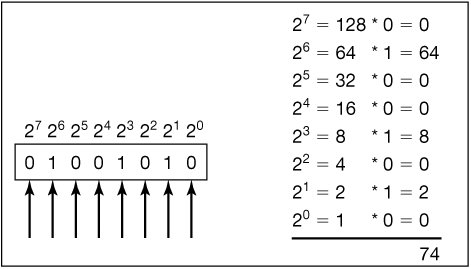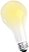# Section 1.2. What Computers Understand

[Page 7]

### 1.2. What Computers Understand

Programs are written to run on computers. What does a computer know how to do? What can we tell the computer to do in the program? The answer is "Very, very little." Computers are exceedingly stupid. They really only know about numbers.

Actually, even to say that computers know numbers is a myth. It might be more appropriate to say that computers are used to encode (represent) numbers. Computers are electronic devices that react to voltages on wires. We group these wires into sets (a set of eight of these wires is called a byte and one wire is called a bit). If a wire has a voltage on it, we say that it encodes a 1. If it has no voltage on it, we say that it encodes a 0. So, from a set of eight wires (a byte), we get a pattern of eight 0's and 1's, e.g., 01001010. Using the binary number system, we can interpret this byte as a decimal number (Figure 1.3). That's where we come up with the claim that a computer knows about numbers.

##### Figure 1.3. Eight wires with a pattern of voltages is a byte, which gets interpreted as a pattern of eight 0's and 1's, which gets interpreted as a decimal number.## Computer Science Idea: Binary Number System

Binary numbers are made up of only 2 digits (0 and 1). We usually work in the decimal number system which has the digits (0 to 9). The value of a decimal number is calculated by multiplying each digit by a power of 10 and summing the result. The powers of 10 start at 0 and increase from right to left. The value of a binary number is calculated by multiplying each digit by a power of 2 and summing the result (Figure 1.3).

The computer has a memory filled with bytes. Everything that a computer is working with at a given instant is stored in its memory. That means that everything that a computer is working with is encoded in its bytes: JPEG pictures, Excel spreadsheets, Word documents, annoying Web pop-up ads, and the latest spam email.

A computer can do lots of things with numbers. It can add them, subtract them, multiply them, divide them, sort them, collect them, duplicate them, filter them (e.g., "make a copy of these numbers, but only the even ones"), and compare them and do things based on the comparison. For example, a computer can be told in a program "Compare these two numbers. If the first one is less than the second one, jump to step 5 in this program. Otherwise, continue on to the next step."

[Page 8]

It sounds like computers are incredible calculators, and that's certainly why they were invented. The first use of computers was during World War II for calculating trajectories of projectiles ("If the wind is coming from the SE at 15 MPH, and you want to hit a target 0.5 miles away at an angle of 30 degrees East of North, then incline your launcher to ..."). The computer is an amazing calculator. But what makes it useful for general programs is the concept of encodings.## Computer Science Idea: Computers can Layer Encodings

Computers can layer encodings to virtually any level of complexity. Numbers can be interpreted as characters, which can be interpreted in groups as Web pages. But at the bottommost level, the computer only "knows" voltages which we interpret as numbers.

If one of these bytes is interpreted as the number 65, it could just be the number 65. Or it could be the letter A using a standard encoding of numbers-to-letters called the American Standard Code for Information Interchange (ASCII). If that 65 appears in a collection of other numbers that we're interpreting as text, and that's in a file that ends in ".html" it might be part of something that looks like this <a href=..., which a Web browser will interpret as the definition of a link. Down at the level of the computer, that A is just a pattern of voltages. Many layers of programs up, at the level of a Web browser, it defines something that you can click on to get more information.

If the computer understands only numbers (and that's a stretch already), how does it manipulate these encodings? Sure, it knows how to compare numbers, but how does that extend to being able to alphabetize a class list? Typically, each layer of encoding is implemented as a piece or layer of software. There's software that understands how to manipulate characters. The character software knows how to do things like compare names because it has encoded that a comes before b and so on, and that the numeric comparison of the order of numbers in the encoding of the letters leads to alphabetical comparisons. The character software is used by other software that manipulates text in files. That's the layer that something like Microsoft Word or Notepad or TextEdit would use. Still another piece of software knows how to interpret HTML (the language of the Web), and another layer of that software knows how to take HTML and display the right text, fonts, styles, and colors.

We can similarly create layers of encodings in the computer for our specific tasks. We can teach a computer that cells contain mitochondria and DNA, and that DNA has four kinds of nucleotides, and that factories have these kinds of presses and these kinds of stamps. Creating layers of encoding and interpretation so that the computer is working with the right units (recall back to our recipe analogy) for a given problem is the task of data representation or defining the right data structures.

If this sounds like lots of software, it is. When software is layered like this, it slows the computer down somewhat. But the amazing thing about computers is that they're amazingly fastand getting faster all the time!

[Page 9]## Computer Science Idea: Moore's Law

Gordon Moore, one of the founders of Intel (maker of computer processing chips for computers running the Windows operating systems), made the claim that the number of transistors (a key component of computers) would double at the same price every 18 months, effectively meaning that the same amount of money would buy twice as much computing power every 18 months. This law has continued to hold true for decades.

Computers today can execute literally BILLIONS of program steps per second! They can hold in memory entire encyclopedias of data! They never get tired nor bored. Search a million customers for a particular card holder? No problem! Find the right set of numbers to get the best value out of an equation? Piece of cake!

Process millions of picture elements or sound fragments or movie frames? That's media computation.Introduction to Computing & Programming Algebra in Java(c) A Multimedia Approach
ISBN: N/A
EAN: N/A
Year: 2007
Pages: 191